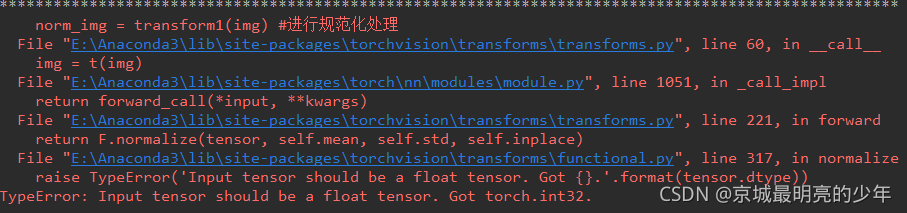# Normalize error: TypeError: Input tensor should be a float tensor…

The following error is reported when using tensor ` normalization``````from torchvision import transforms
import numpy as np
import torchvision
import torch

data = np.random.randint(0, 255, size=12)
img = data.reshape(2,2,3)

print(img)
print("*"*100)
transform1 = transforms.Compose([
transforms.ToTensor(), # range [0, 255] -> [0.0,1.0]
transforms.Normalize(mean = (10,10,10), std = (1,1,1)),
]
)
# img = img.astype('float')
norm_img = transform1(img)
print(norm_img)

``````

You can add this sentence. In fact, it is to set the element type. See the tips above